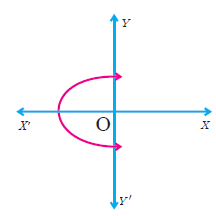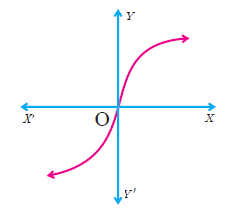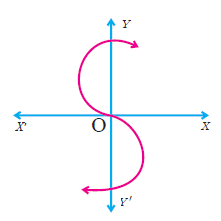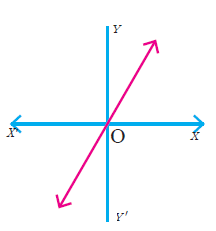## Pages

### Determine whether the graph given below represent functions. Give reasons for your answers concerning each graph.Solution :
Since the graph intersects the vertical line (y-axis) at two points, it is not a function.Solution :
The given graph intersects the vertical line (y-axis) at one point. It is a function.
(iii)Solution :
The graph intersects the y-axis at three points, hence it is not a function.Solution :
The graph intersects the vertical line at most one point. Hence it is a function.